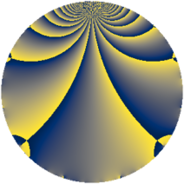# Properties

 Label 1815.2.fLevel $1815$ Weight $2$ Character orbit 1815.f Rep. character $\chi_{1815}(1451,\cdot)$ Character field $\Q$ Dimension $144$ Sturm bound $528$

# Related objects

## Defining parameters

 Level: $$N$$ $$=$$ $$1815 = 3 \cdot 5 \cdot 11^{2}$$ Weight: $$k$$ $$=$$ $$2$$ Character orbit: $$[\chi]$$ $$=$$ 1815.f (of order $$2$$ and degree $$1$$) Character conductor: $$\operatorname{cond}(\chi)$$ $$=$$ $$33$$ Character field: $$\Q$$ Sturm bound: $$528$$

## Dimensions

The following table gives the dimensions of various subspaces of $$M_{2}(1815, [\chi])$$.

Total New Old
Modular forms 288 144 144
Cusp forms 240 144 96
Eisenstein series 48 0 48

## Trace form

 $$144q + 4q^{3} + 144q^{4} - 8q^{9} + O(q^{10})$$ $$144q + 4q^{3} + 144q^{4} - 8q^{9} + 12q^{12} + 4q^{15} + 176q^{16} - 144q^{25} - 20q^{27} + 24q^{34} + 44q^{36} - 24q^{37} - 12q^{42} + 32q^{48} - 152q^{49} - 12q^{60} + 160q^{64} - 88q^{67} + 40q^{69} - 24q^{70} - 4q^{75} - 92q^{78} + 64q^{82} + 56q^{91} + 72q^{93} - 32q^{97} + O(q^{100})$$

## Decomposition of $$S_{2}^{\mathrm{new}}(1815, [\chi])$$ into newform subspaces

The newforms in this space have not yet been added to the LMFDB.

## Decomposition of $$S_{2}^{\mathrm{old}}(1815, [\chi])$$ into lower level spaces

$$S_{2}^{\mathrm{old}}(1815, [\chi]) \cong$$ $$S_{2}^{\mathrm{new}}(33, [\chi])$$$$^{\oplus 4}$$$$\oplus$$$$S_{2}^{\mathrm{new}}(165, [\chi])$$$$^{\oplus 2}$$$$\oplus$$$$S_{2}^{\mathrm{new}}(363, [\chi])$$$$^{\oplus 2}$$